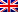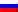# Descent methods

Contenus

## Descent methods

As the explicit name indicates, the methods of descent, or local search, consist in "sliding" along the objective function until a local optimum is found, that is to say where the descent is no longer possible. There are several kinds of descent method which we will describe here.

## Simple descent

From an initial solution, the simple descent algorithm chooses a neighboring solution better than the current solution, until it cannot make such a choice.

The algorithm is as follows:

```S_O
to do
S(i + 1) = neighbor (Si)
if f (S(i + 1)) <f (Si) accept S(i + 1)
end if
as long as f (S(i + 1)) <f (Si), whatever S(i + 1) = neighbor (Si)
return Rnot```

## Greatest descent

The algorithm is mainly the same as for the simple descent, only a selection criterion of a neighboring solution is modified:

`choose s' neighbor of s / f (s') <f (s' ') or f (s') = f (s'') qq is s' 'neighbor of s`

We therefore choose the neighboring solution offering the best improvement of the current solution.

## Multi-start descent

Multi-start descent performs multiple instances of the single descent or greater descent problem. The algorithm is as follows:

```iter = 1 f (Best) = infinity
to do
Choose a starting solution sO at random s 0)
if f (s) <f(Best) alors Best tant que iter < iterMax
return Bes```

##FRFRENRUES